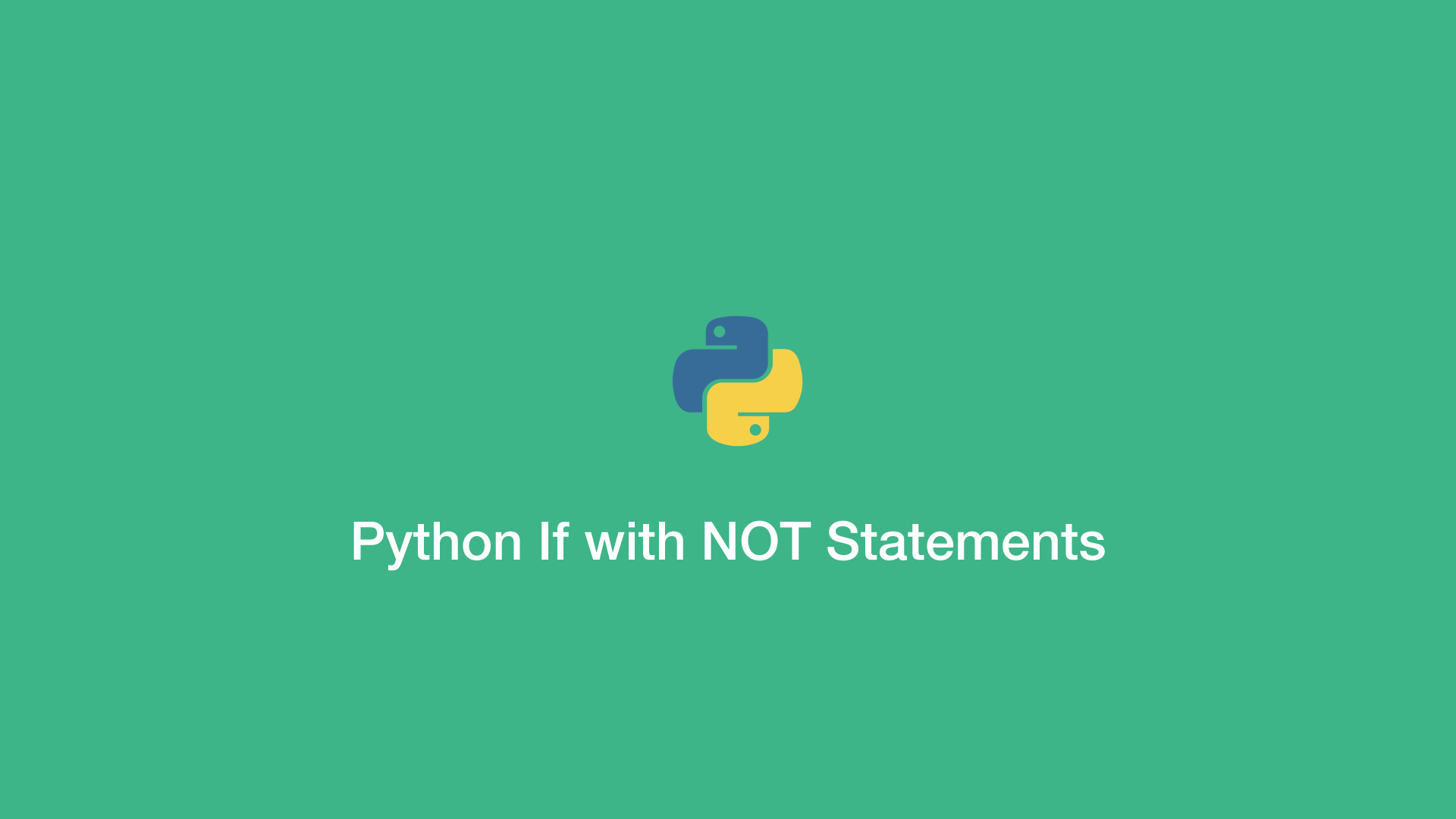# Python If with NOT StatementsAn `if NOT` statement in Python will only execute code inside the block if the boolean value supplied is `False`, or an iterable supplied is empty.

In this tutorial, we will cover how to use `if NOT` statements in Python for a variety of different data types such as, boolean, string, list, dictionary and tuple.

## Python if not Boolean Example

``````item = False

if not item:
print('Boolean value is false.')
``````
``````Boolean value is false.
``````

## Python if not String Example

If the string supplied to an `if NOT` statement is empty, the code will run inside the block:

``````item = ''

if not item:
print('String is empty, do something here.')
``````
``````String is empty, do something here.
``````

## Python if not List Example

An `if NOT` statement is a good way to evaluate if a list is empty and execute some code if that is the case:

``````item = []

if not item:
print('List is empty, do something here.')
``````
``````List is empty, do something here.
``````

## Python if not Dictionary Example

You can check whether a Python dictionary is empty and run code like this:

``````item = dict({})

if not item:
print('Dictionary is empty, do something here.')
``````
``````Dictionary is empty, do something here.
``````

The same above also applies to empty sets and tuples. If they are empty, an `if NOT` statement will execute code inside the block.

``````item1 = set({})

item2 = tuple()

if not item1:
print('Set is empty, do something here.')

if not item2:
print('Tuple is empty, do something here.')
``````
``````Set is empty, do something here.
Tuple is empty, do something here.
``````

#### Related Tutorials### How to Use if and else Statements in Python

August 31, 2020### Python Assert Statement

September 30, 2020### How to use Python Try Except Block

June 14, 2021### How to Compare Strings in Python

September 20, 2020### How to Check if a String Contains a Substring in Python

September 21, 2020October 04, 2020